Ex 3.2

Chapter 3 Class 10 Pair of Linear Equations in Two Variables
Serial order wise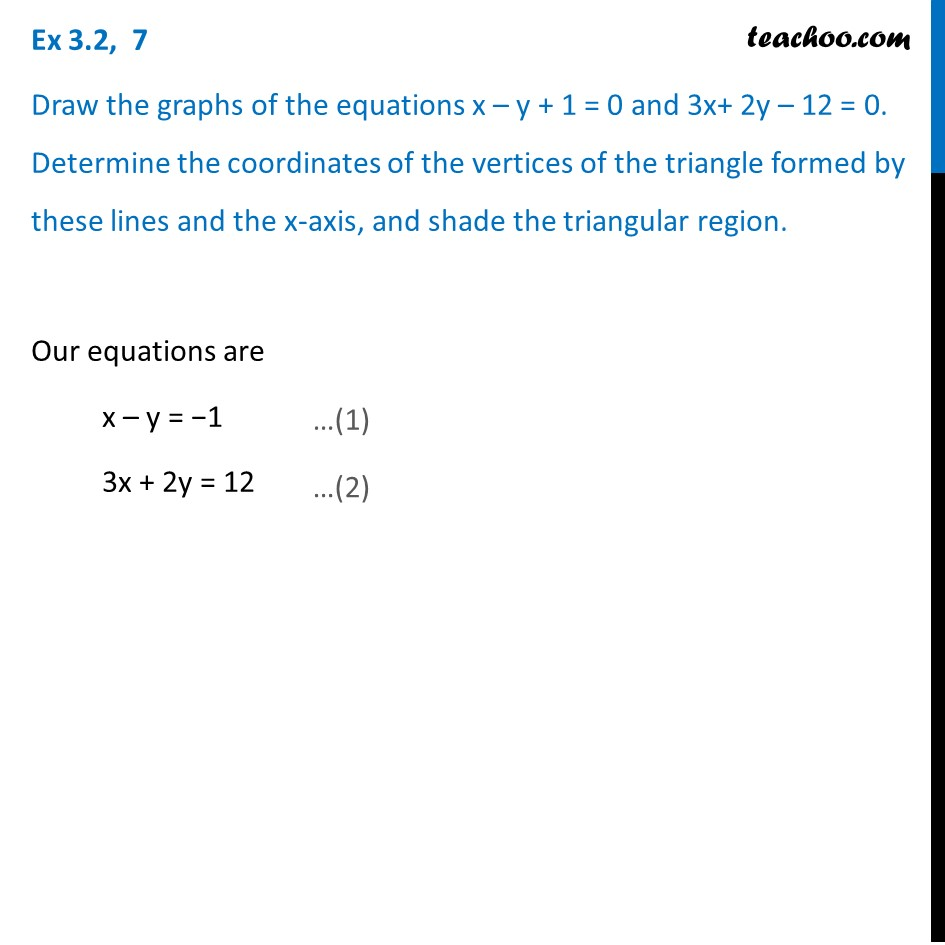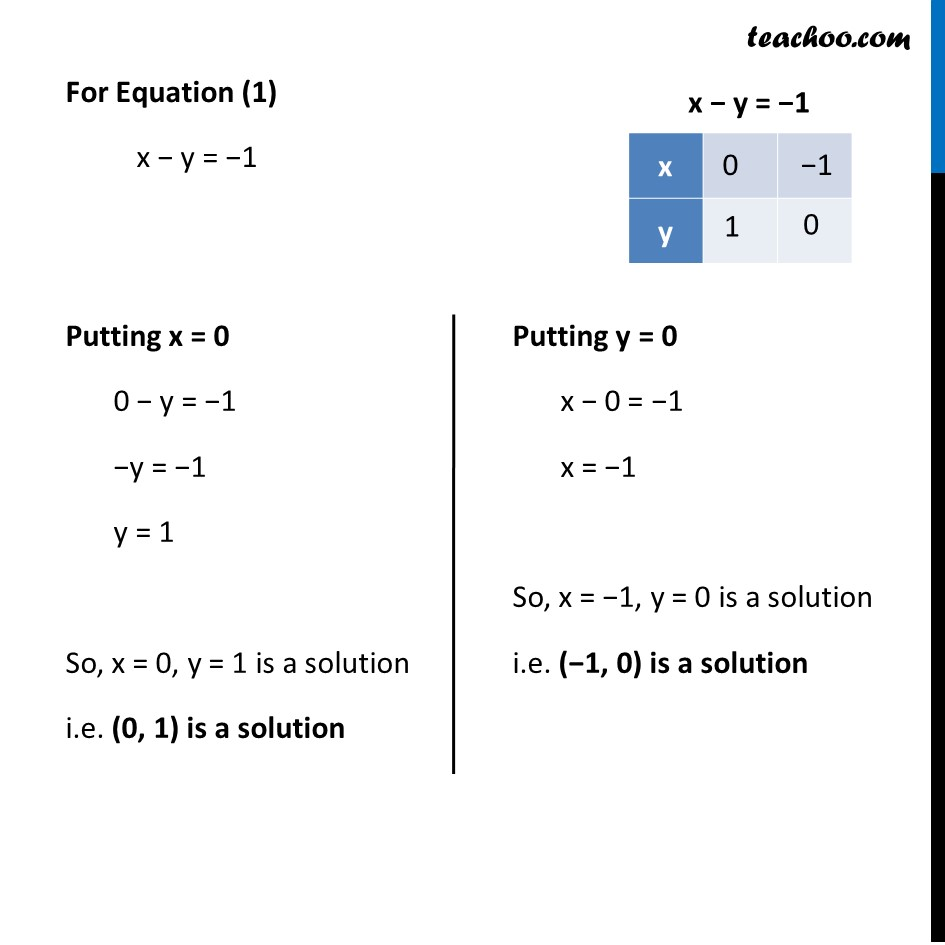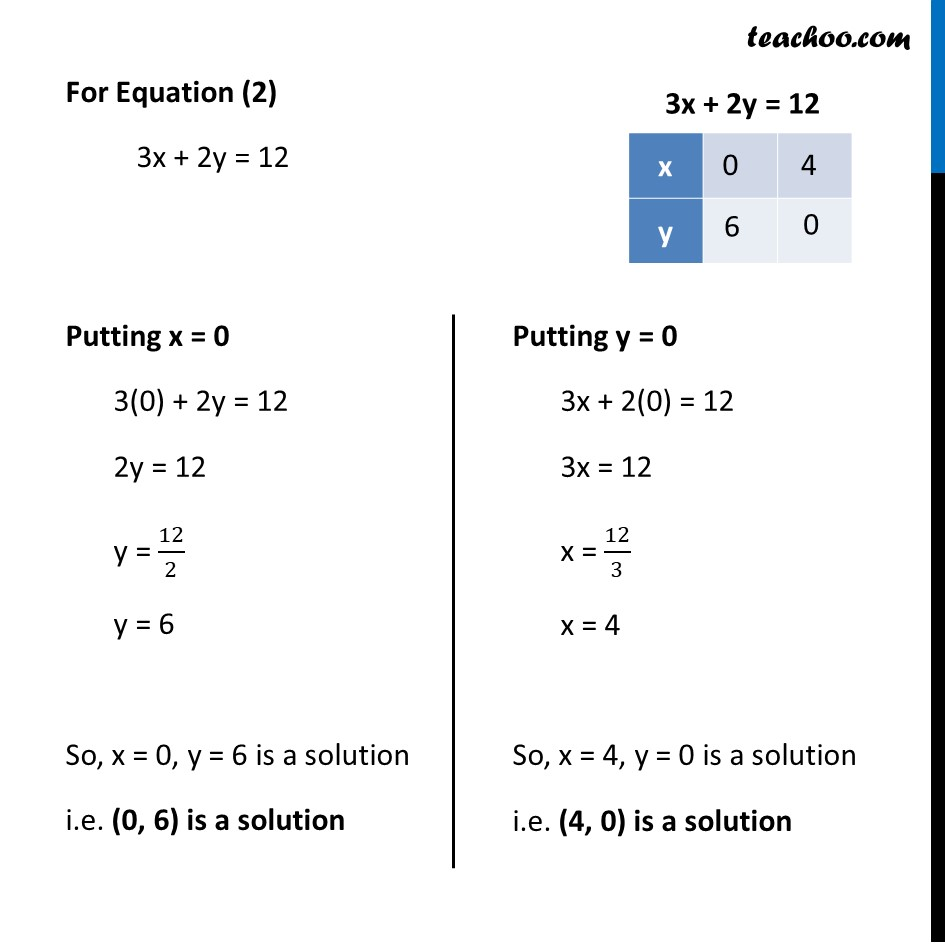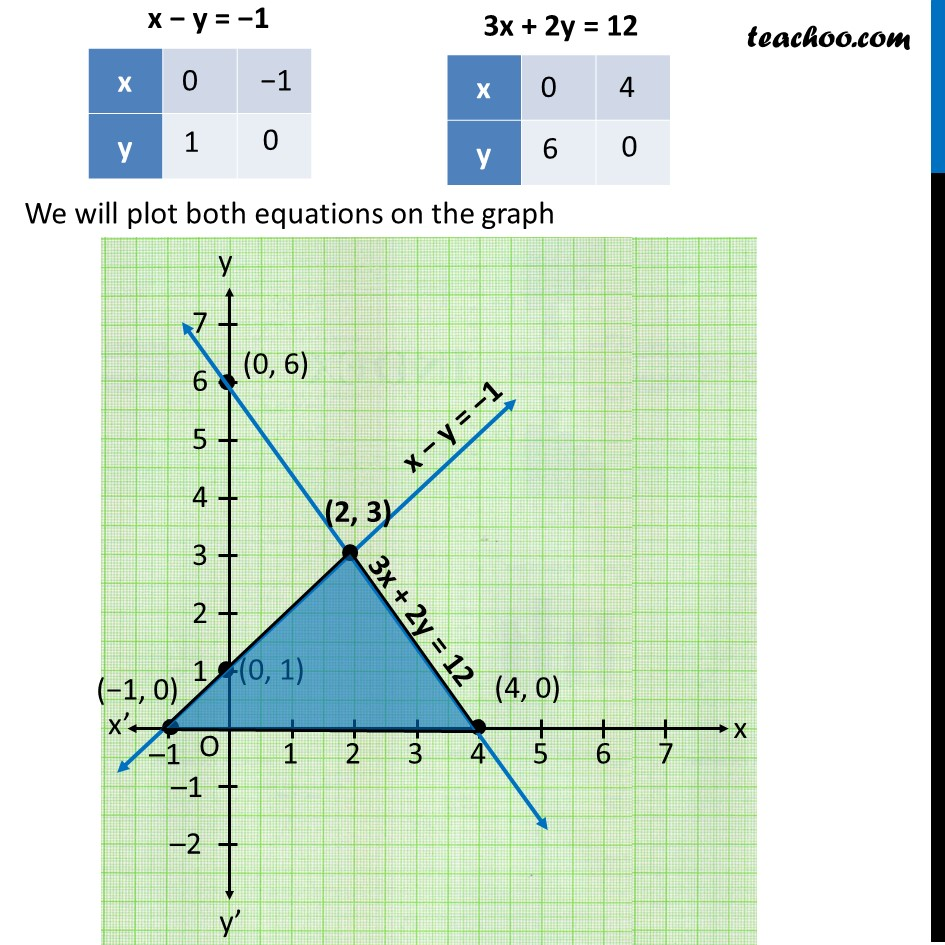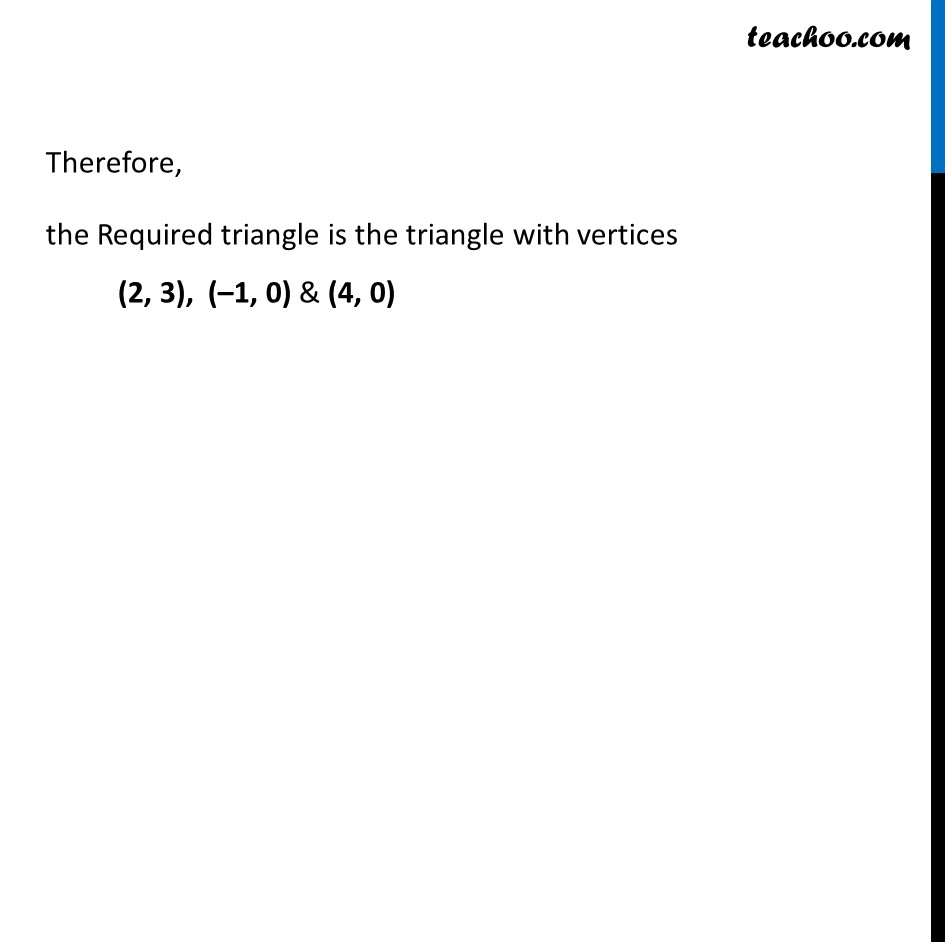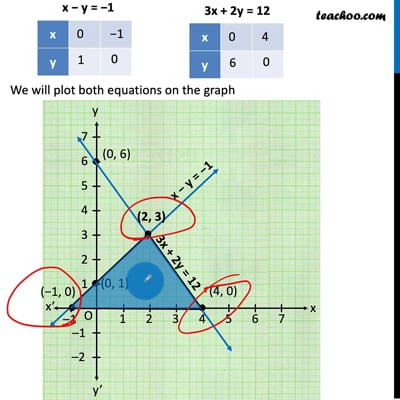This video is only available for Teachoo black users

Solve all your doubts with Teachoo Black (new monthly pack available now!)

### Transcript

Ex 3.2, 7 Draw the graphs of the equations x – y + 1 = 0 and 3x+ 2y – 12 = 0. Determine the coordinates of the vertices of the triangle formed by these lines and the x-axis, and shade the triangular region. Our equations are x – y = −1 3x + 2y = 12 For Equation (1) x − y = −1 Putting x = 0 0 − y = −1 −y = −1 y = 1 So, x = 0, y = 1 is a solution i.e. (0, 1) is a solution Putting y = 0 x − 0 = −1 x = −1 So, x = −1, y = 0 is a solution i.e. (−1, 0) is a solution For Equation (2) 3x + 2y = 12 Putting x = 0 3(0) + 2y = 12 2y = 12 y = 12/2 y = 6 So, x = 0, y = 6 is a solution i.e. (0, 6) is a solution Putting y = 0 3x + 2(0) = 12 3x = 12 x = 12/3 x = 4 So, x = 4, y = 0 is a solution i.e. (4, 0) is a solution We will plot both equations on the graph Therefore, the Required triangle is the triangle with vertices (2, 3), (–1, 0) & (4, 0)